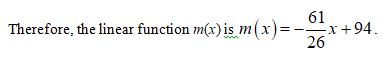# Algeria is a developing country on the northern coast of Africa. Its infant mortality rates (number of deaths per 1000 live births) declined from 94 in 1980 to 33 in 2006.a. Assume that the infant mortality rate is declining linearly over time. Construct an equation modeling the relationship between infant mortality rate and time, where time is measured in years since 1980.Round any calculations to two decimal places.d(x)= , where d(x) is the number of deaths per 1000 live births x years from 1980.b. Assuming that the infant mortality rate is declining exponentially over time, construct an equation modeling the relationship, where time is measured in years since 1980.Round any calculations to four decimal places.m(x)= , where m(x) is the number of deaths per 1000 live births x years from 1980.c. What would each of your models predict for the infant mortality rate in 2011?Round your answers to the nearest integer.Using the linear model,  deaths per 1000 live births in 2011.Using the exponential model,  deaths per 1000 live births in 2011.

Question

Algeria is a developing country on the northern coast of Africa. Its infant mortality rates (number of deaths per 1000 live births) declined from 94 in 1980 to 33 in 2006.

a. Assume that the infant mortality rate is declining linearly over time. Construct an equation modeling the relationship between infant mortality rate and time, where time is measured in years since 1980.

Round any calculations to two decimal places.

d(x)=

, where d(x) is the number of deaths per 1000 live births x years from 1980.

b. Assuming that the infant mortality rate is declining exponentially over time, construct an equation modeling the relationship, where time is measured in years since 1980.

Round any calculations to four decimal places.

m(x)=

, where m(x) is the number of deaths per 1000 live births x years from 1980.

c. What would each of your models predict for the infant mortality rate in 2011?

Using the linear model,  deaths per 1000 live births in 2011.

Using the exponential model,  deaths per 1000 live births in 2011.

check_circleExpert Solution
Step 1

(a) It is given that the infant mortality rate declined from 94 in 1980 to 33 in 2006 .Let m(x) represent number of infant mortality rate declined and x be the number of years since 1980.Let the coordinate points be (0,94) and (26,33).Obtain m(x)  as a linear function of x.

Step 2

Use the slope and the point (0,94) to find the linear function of x.

Step 3...

### Want to see the full answer?

See Solution

#### Want to see this answer and more?

Solutions are written by subject experts who are available 24/7. Questions are typically answered within 1 hour*

See Solution
*Response times may vary by subject and question
Tagged in

### Algebra